## Imaging Pipeline

This section discusses the complete Imaging Pipeline: the pixel-storage modes and pixel-transfer operations, which include how to set up an arbitrary mapping to convert pixel data. You can also magnify or reduce a pixel rectangle before it's drawn by calling glPixelZoom(). The order of these operations is shown in Figure 8-4.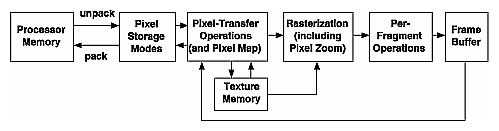Figure 8-4 : Imaging Pipeline

When glDrawPixels() is called, the data is first unpacked from processor memory according to the pixel-storage modes that are in effect and then the pixel-transfer operations are applied. The resulting pixels are then rasterized. During rasterization, the pixel rectangle may be zoomed up or down, depending on the current state. Finally, the fragment operations are applied and the pixels are written into the framebuffer. (See "Testing and Operating on Fragments" in Chapter 10 for a discussion of the fragment operations.)

When glReadPixels() is called, data is read from the framebuffer, the pixel-transfer operations are performed, and then the resulting data is packed into processor memory.

glCopyPixels() applies all the pixel-transfer operations during what would be the glReadPixels() activity. The resulting data is written as it would be by glDrawPixels(), but the transformations aren't applied a second time. Figure 8-5 shows how glCopyPixels() moves pixel data, starting from the frame buffer.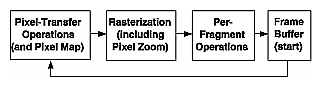Figure 8-5 : glCopyPixels() Pixel Path

From "Drawing the Bitmap" and Figure 8-6, you see that rendering bitmaps is simpler than rendering images. Neither the pixel-transfer operations nor the pixel-zoom operation are applied.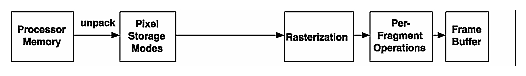Figure 8-6 : glBitmap() Pixel Path

Note that the pixel-storage modes and pixel-transfer operations are applied to textures as they are read from or written to texture memory. Figure 8-7 shows the effect on glTexImage*(), glTexSubImage*(), and glGetTexImage().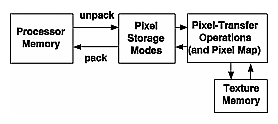Figure 8-7 : glTexImage*(), glTexSubImage*(), and glGetTexImage() Pixel Paths

As seen in Figure 8-8, when pixel data is copied from the framebuffer into texture memory (glCopyTexImage*() or glCopyTexSubImage*()), only pixel-transfer operations are applied. (See Chapter 9 for more information on textures.)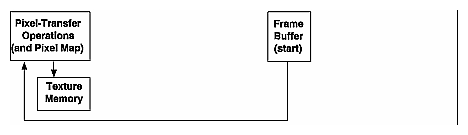Figure 8-8 : glCopyTexImage*() and glCopyTexSubImage*() Pixel Paths

### Pixel Packing and Unpacking

Packing and unpacking refer to the way that pixel data is written to and read from processor memory.

An image stored in memory has between one and four chunks of data, called elements. The data might consist of just the color index or the luminance (luminance is the weighted sum of the red, green, and blue values), or it might consist of the red, green, blue, and alpha components for each pixel. The possible arrangements of pixel data, or formats, determine the number of elements stored for each pixel and their order.

Some elements (such as a color index or a stencil index) are integers, and others (such as the red, green, blue, and alpha components, or the depth component) are floating-point values, typically ranging between 0.0 and 1.0. Floating-point components are usually stored in the framebuffer with lower resolution than a full floating-point number would require (for example, color components may be stored in 8 bits). The exact number of bits used to represent the components depends on the particular hardware being used. Thus, it's often wasteful to store each component as a full 32-bit floating-point number, especially since images can easily contain a million pixels.

Elements can be stored in memory as various data types, ranging from 8-bit bytes to 32-bit integers or floating-point numbers. OpenGL explicitly defines the conversion of each component in each format to each of the possible data types. Keep in mind that you may lose data if you try to store a high-resolution component in a type represented by a small number of bits.

### Controlling Pixel-Storage Modes

Image data is typically stored in processor memory in rectangular two- or three-dimensional arrays. Often, you want to display or store a subimage that corresponds to a subrectangle of the array. In addition, you might need to take into account that different machines have different byte-ordering conventions. Finally, some machines have hardware that is far more efficient at moving data to and from the framebuffer if the data is aligned on 2-byte, 4-byte, or 8-byte boundaries in processor memory. For such machines, you probably want to control the byte alignment. All the issues raised in this paragraph are controlled as pixel-storage modes, which are discussed in the next subsection. You specify these modes by using glPixelStore*(), which you've already seen used in a couple of example programs.

All the possible pixel-storage modes are controlled with the glPixelStore*() command. Typically, several successive calls are made with this command to set several parameter values.

void glPixelStore{if}(GLenum pname, TYPEparam);

Sets the pixel-storage modes, which affect the operation of glDrawPixels(), glReadPixels(), glBitmap(), glPolygonStipple(), glTexImage1D(), glTexImage2D(), glTexSubImage1D(), glTexSubImage2D(), and glGetTexImage(). The possible parameter names for pname are shown in Table 8-3, along with their data type, initial value, and valid range of values. The GL_UNPACK* parameters control how data is unpacked from memory by glDrawPixels(), glBitmap(), glPolygonStipple(), glTexImage1D(), glTexImage2D(), glTexSubImage1D(), and glTexSubImage2D(). The GL_PACK* parameters control how data is packed into memory by glReadPixels() and glGetTexImage().

 Table 8-3 : glPixelStore() Parameters Parameter Name Type Initial Value Valid Range GL_UNPACK_SWAP_BYTES, GL_PACK_SWAP_BYTES GLboolean FALSE TRUE/FALSE GL_UNPACK_LSB_FIRST, GL_PACK_LSB_FIRST GLboolean FALSE TRUE/FALSE GL_UNPACK_ROW_LENGTH, GL_PACK_ROW_LENGTH GLint 0 any nonnegative integer GL_UNPACK_SKIP_ROWS, GL_PACK_SKIP_ROWS GLint 0 any nonnegative integer GL_UNPACK_SKIP_PIXELS, GL_PACK_SKIP_PIXELS GLint 0 any nonnegative integer GL_UNPACK_ALIGNMENT, GL_PACK_ALIGNMENT GLint 4 1, 2, 4, 8

Since the corresponding parameters for packing and unpacking have the same meanings, they're discussed together in the rest of this section and referred to without the GL_PACK or GL_UNPACK prefix. For example, *SWAP_BYTES refers to GL_PACK_SWAP_BYTES and GL_UNPACK_SWAP_BYTES.

If the *SWAP_BYTES parameter is FALSE (the default), the ordering of the bytes in memory is whatever is native for the OpenGL client; otherwise, the bytes are reversed. The byte reversal applies to any size element, but really only has a meaningful effect for multibyte elements.

Note: As long as your OpenGL application doesn't share images with other machines, you can ignore the issue of byte ordering. If your application must render an OpenGL image that was created on a different machine and the "endianness" of the two machines differs, byte ordering can be swapped using *SWAP_BYTES. However, *SWAP_BYTES does not allow you to reorder elements (for example, to swap red and green).

The *LSB_FIRST parameter applies when drawing or reading 1-bit images or bitmaps, for which a single bit of data is saved or restored for each pixel. If *LSB_FIRST is FALSE (the default), the bits are taken from the bytes starting with the most significant bit; otherwise, they're taken in the opposite order. For example, if *LSB_FIRST is FALSE, and the byte in question is 0x31, the bits, in order, are {0, 0, 1, 1, 0, 0, 0, 1}. If *LSB_FIRST is TRUE, the order is {1, 0, 0, 0, 1, 1, 0, 0}.

Sometimes you want to draw or read only a subrectangle of the entire rectangle of image data stored in memory. If the rectangle in memory is larger than the subrectangle that's being drawn or read, you need to specify the actual length (measured in pixels) of the larger rectangle with *ROW_LENGTH. If *ROW_LENGTH is zero (which it is by default), the row length is understood to be the same as the width that's specified with glReadPixels(), glDrawPixels(), or glCopyPixels(). You also need to specify the number of rows and pixels to skip before starting to copy the data for the subrectangle. These numbers are set using the parameters *SKIP_ROWS and *SKIP_PIXELS, as shown in Figure 8-9. By default, both parameters are 0, so you start at the lower-left corner.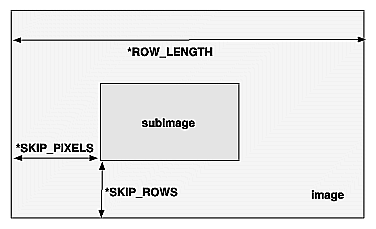Figure 8-9 : *SKIP_ROWS, *SKIP_PIXELS, and *ROW_LENGTH Parameters

Often a particular machine's hardware is optimized for moving pixel data to and from memory, if the data is saved in memory with a particular byte alignment. For example, in a machine with 32-bit words, hardware can often retrieve data much faster if it's initially aligned on a 32-bit boundary, which typically has an address that is a multiple of 4. Likewise, 64-bit architectures might work better when the data is aligned to 8-byte boundaries. On some machines, however, byte alignment makes no difference.

As an example, suppose your machine works better with pixel data aligned to a 4-byte boundary. Images are most efficiently saved by forcing the data for each row of the image to begin on a 4-byte boundary. If the image is 5 pixels wide and each pixel consists of 1 byte each of red, green, and blue information, a row requires 5 × 3 = 15 bytes of data. Maximum display efficiency can be achieved if the first row, and each successive row, begins on a 4-byte boundary, so there is 1 byte of waste in the memory storage for each row. If your data is stored like this, set the *ALIGNMENT parameter appropriately (to 4, in this case).

If *ALIGNMENT is set to 1, the next available byte is used. If it's 2, a byte is skipped if necessary at the end of each row so that the first byte of the next row has an address that's a multiple of 2. In the case of bitmaps (or 1-bit images) where a single bit is saved for each pixel, the same byte alignment works, although you have to count individual bits. For example, if you're saving a single bit per pixel, the row length is 75, and the alignment is 4, then each row requires 75/8, or 9 3/8 bytes. Since 12 is the smallest multiple of 4 that is bigger than 9 3/8, 12 bytes of memory are used for each row. If the alignment is 1, then 10 bytes are used for each row, as 9 3/8 is rounded up to the next byte. (There is a simple use of glPixelStorei() in Example 8-4.)

### Pixel-Transfer Operations

As image data is transferred from memory into the framebuffer, or from the framebuffer into memory, OpenGL can perform several operations on it. For example, the ranges of components can be altered - normally, the red component is between 0.0 and 1.0, but you might prefer to keep it in some other range; or perhaps the data you're using from a different graphics system stores the red component in a different range. You can even create maps to perform arbitrary conversion of color indices or color components during pixel transfer. Conversions such as these performed during the transfer of pixels to and from the framebuffer are called pixel-transfer operations. They're controlled with the glPixelTransfer*() and glPixelMap*() commands.

Be aware that although the color, depth, and stencil buffers have many similarities, they don't behave identically, and a few of the modes have special cases for special buffers. All the mode details are covered in this section and the sections that follow, including all the special cases.

Some of the pixel-transfer function characteristics are set with glPixelTransfer*(). The other characteristics are specified with glPixelMap*(), which is described in the next section.

void glPixelTransfer{if}(GLenum pname, TYPEparam);

Sets pixel-transfer modes that affect the operation of glDrawPixels(), glReadPixels(), glCopyPixels(), glTexImage1D(), glTexImage2D(), glCopyTexImage1D(), glCopyTexImage2D(), glTexSubImage1D(), glTexSubImage2D(), glCopyTexSubImage1D(), glCopyTexSubImage2D(), and glGetTexImage(). The parameter pname must be one of those listed in the first column of Table 8-4, and its value, param, must be in the valid range shown.

 Table 8-4 : glPixelTransfer*() Parameters (continued) Parameter Name Type Initial Value Valid Range GL_MAP_COLOR GLboolean FALSE TRUE/FALSE GL_MAP_STENCIL GLboolean FALSE TRUE/FALSE GL_INDEX_SHIFT GLint 0 (- ∞ , ∞ ) GL_INDEX_OFFSET GLint 0 (- ∞ , ∞ ) GL_RED_SCALE GLfloat 1.0 (- ∞ , ∞ ) GL_GREEN_SCALE GLfloat 1.0 (- ∞ , ∞ ) GL_BLUE_SCALE GLfloat 1.0 (- ∞ , ∞ ) GL_ALPHA_SCALE GLfloat 1.0 (- ∞ , ∞ ) GL_DEPTH_SCALE GLfloat 1.0 (- ∞ , ∞ ) GL_RED_BIAS GLfloat 0 (- ∞ , ∞ ) GL_GREEN_BIAS GLfloat 0 (- ∞ , ∞ ) GL_BLUE_BIAS GLfloat 0 (- ∞ , ∞ ) GL_ALPHA_BIAS GLfloat 0 (- ∞ , ∞ ) GL_DEPTH_BIAS GLfloat 0 (- ∞ , ∞ )

If the GL_MAP_COLOR or GL_MAP_STENCIL parameter is TRUE, then mapping is enabled. See the next subsection to learn how the mapping is done and how to change the contents of the maps. All the other parameters directly affect the pixel component values.

A scale and bias can be applied to the red, green, blue, alpha, and depth components. For example, you may wish to scale red, green, and blue components that were read from the framebuffer before converting them to a luminance format in processor memory. Luminance is computed as the sum of the red, green, and blue components, so if you use the default value for GL_RED_SCALE, GL_GREEN_SCALE and GL_BLUE_SCALE, the components all contribute equally to the final intensity or luminance value. If you want to convert RGB to luminance, according to the NTSC standard, you set GL_RED_SCALE to .30, GL_GREEN_SCALE to .59, and GL_BLUE_SCALE to .11.

Indices (color and stencil) can also be transformed. In the case of indices a shift and offset are applied. This is useful if you need to control which portion of the color table is used during rendering.

### Pixel Mapping

All the color components, color indices, and stencil indices can be modified by means of a table lookup before they are placed in screen memory. The command for controlling this mapping is glPixelMap*().

void glPixelMap{ui us f}v(GLenum map, GLint mapsize,
const TYPE *values);

Loads the pixel map indicated by map with mapsize entries, whose values are pointed to by values. Table 8-5 lists the map names and values; the default sizes are all 1 and the default values are all 0. Each map's size must be a power of 2.

 Table 8-5 : glPixelMap*() Parameter Names and Values Map Name Address Value GL_PIXEL_MAP_I_TO_I color index color index GL_PIXEL_MAP_S_TO_S stencil index stencil index GL_PIXEL_MAP_I_TO_R color index R GL_PIXEL_MAP_I_TO_G color index G GL_PIXEL_MAP_I_TO_B color index B GL_PIXEL_MAP_I_TO_A color index A GL_PIXEL_MAP_R_TO_R R R GL_PIXEL_MAP_G_TO_G G G GL_PIXEL_MAP_B_TO_B B B GL_PIXEL_MAP_A_TO_A A A

The maximum size of the maps is machine-dependent. You can find the sizes of the pixel maps supported on your machine with glGetIntegerv(). Use the query argument GL_MAX_PIXEL_MAP_TABLE to obtain the maximum size for all the pixel map tables, and use GL_PIXEL_MAP_*_TO_*_SIZE to obtain the current size of the specified map. The six maps whose address is a color index or stencil index must always be sized to an integral power of 2. The four RGBA maps can be any size from 1 through GL_MAX_PIXEL_MAP_TABLE.

To understand how a table works, consider a simple example. Suppose that you want to create a 256-entry table that maps color indices to color indices using GL_PIXEL_MAP_I_TO_I. You create a table with an entry for each of the values between 0 and 255 and initialize the table with glPixelMap*(). Assume you're using the table for thresholding and want to map indices below 101 (indices 0 to 100) to 0, and all indices 101 and above to 255. In this case, your table consists of 101 0s and 155 255s. The pixel map is enabled using the routine glPixelTransfer*() to set the parameter GL_MAP_COLOR to TRUE. Once the pixel map is loaded and enabled, incoming color indices below 101 come out as 0, and incoming pixels between 101 and 255 are mapped to 255. If the incoming pixel is larger than 255, it's first masked by 255, throwing out all the bits above the eighth, and the resulting masked value is looked up in the table. If the incoming index is a floating-point value (say 88.14585), it's rounded to the nearest integer value (giving 88), and that number is looked up in the table (giving 0).

Using pixel maps, you can also map stencil indices or convert color indices to RGB. (See "Reading and Drawing Pixel Rectangles" for information about the conversion of indices.)

### Magnifying, Reducing, or Flipping an Image

After the pixel-storage modes and pixel-transfer operations are applied, images and bitmaps are rasterized. Normally, each pixel in an image is written to a single pixel on the screen. However, you can arbitrarily magnify, reduce, or even flip (reflect) an image by using glPixelZoom().

void glPixelZoom(GLfloat zoomx, GLfloat zoomy);

Sets the magnification or reduction factors for pixel-write operations (glDrawPixels() or glCopyPixels()), in the x- and y-dimensions. By default, zoomx and zoomy are 1.0. If they're both 2.0, each image pixel is drawn to 4 screen pixels. Note that fractional magnification or reduction factors are allowed, as are negative factors. Negative zoom factors reflect the resulting image about the current raster position.

During rasterization, each image pixel is treated as a zoomx × zoomy rectangle, and fragments are generated for all the pixels whose centers lie within the rectangle. More specifically, let (xrp, yrp) be the current raster position. If a particular group of elements (index or components) is the nth in a row and belongs to the mth column, consider the region in window coordinates bounded by the rectangle with corners at

(xrp + zoomx * n, yrp + zoomy * m) and (xrp + zoomx(n+1), yrp + zoomy(m+1))

Any fragments whose centers lie inside this rectangle (or on its bottom or left boundaries) are produced in correspondence with this particular group of elements.

A negative zoom can be useful for flipping an image. OpenGL describes images from the bottom row of pixels to the top (and from left to right). If you have a "top to bottom" image, such as a frame of video, you may want to use glPixelZoom(1.0, -1.0) to make the image right side up for OpenGL. Be sure that you reposition the current raster position appropriately, if needed.

Example 8-4 shows the use of glPixelZoom(). A checkerboard image is initially drawn in the lower-left corner of the window. Pressing a mouse button and moving the mouse uses glCopyPixels() to copy the lower-left corner of the window to the current cursor location. (If you copy the image onto itself, it looks wacky!) The copied image is zoomed, but initially it is zoomed by the default value of 1.0, so you won't notice. The `z' and `Z' keys increase and decrease the zoom factors by 0.5. Any window damage causes the contents of the window to be redrawn. Pressing the `r' key resets the image and the zoom factors.

Example 8-4 : Drawing, Copying, and Zooming Pixel Data: image.c

`#include <GL/gl.h>`
`#include <GL/glu.h>`
`#include <GL/glut.h>`
`#include <stdlib.h>`
`#include <stdio.h>`
` `
`#define checkImageWidth 64`
`#define checkImageHeight 64`
`GLubyte checkImage[checkImageHeight][checkImageWidth];`
` `
`static GLdouble zoomFactor = 1.0;`
`static GLint height;`
` `
`void makeCheckImage(void)`
`{`
`   int i, j, c;`
`    `
`   for (i = 0; i < checkImageHeight; i++) {`
`      for (j = 0; j < checkImageWidth; j++) {`
`         c = ((((i&0x8)==0)^((j&0x8))==0))*255;`
`         checkImage[i][j] = (GLubyte) c;`
`         checkImage[i][j] = (GLubyte) c;`
`         checkImage[i][j] = (GLubyte) c;`
`      }`
`   }`
`}`
` `
`void init(void)`
`{   `
`   glClearColor (0.0, 0.0, 0.0, 0.0);`
`   glShadeModel(GL_FLAT);`
`   makeCheckImage();`
`   glPixelStorei(GL_UNPACK_ALIGNMENT, 1);`
`}`
` `
`void display(void)`
`{`
`   glClear(GL_COLOR_BUFFER_BIT);`
`   glRasterPos2i(0, 0);`
`   glDrawPixels(checkImageWidth, checkImageHeight, GL_RGB,`
`                GL_UNSIGNED_BYTE, checkImage);`
`   glFlush();`
`}`
` `
`void reshape(int w, int h)`
`{`
`   glViewport(0, 0, (GLsizei) w, (GLsizei) h);`
`   height = (GLint) h;`
`   glMatrixMode(GL_PROJECTION);`
`   glLoadIdentity();`
`   gluOrtho2D(0.0, (GLdouble) w, 0.0, (GLdouble) h);`
`   glMatrixMode(GL_MODELVIEW);`
`   glLoadIdentity();`
`}`
` `
`void motion(int x, int y)`
`{`
`   static GLint screeny;`
`   `
`   screeny = height - (GLint) y;`
`   glRasterPos2i (x, screeny);`
`   glPixelZoom (zoomFactor, zoomFactor);`
`   glCopyPixels (0, 0, checkImageWidth, checkImageHeight,`
`                 GL_COLOR);`
`   glPixelZoom (1.0, 1.0);`
`   glFlush ();`
`}`
` `
`void keyboard(unsigned char key, int x, int y)`
`{`
`   switch (key) {`
`      case `r':`
`      case `R':`
`         zoomFactor = 1.0;`
`         glutPostRedisplay();`
`         printf ("zoomFactor reset to 1.0\n");`
`         break;`
`      case `z':`
`         zoomFactor += 0.5;`
`         if (zoomFactor >= 3.0)`
`            zoomFactor = 3.0;`
`         printf ("zoomFactor is now %4.1f\n", zoomFactor);`
`         break;`
`      case `Z':`
`         zoomFactor -= 0.5;`
`         if (zoomFactor <= 0.5)`
`            zoomFactor = 0.5;`
`         printf ("zoomFactor is now %4.1f\n", zoomFactor);`
`         break;`
`      case 27:`
`         exit(0);`
`         break;`
`      default:`
`         break;`
`   }`
`}`
` `
`int main(int argc, char** argv)`
`{`
`   glutInit(&argc, argv);`
`   glutInitDisplayMode(GLUT_SINGLE | GLUT_RGB);`
`   glutInitWindowSize(250, 250);`
`   glutInitWindowPosition(100, 100);`
`   glutCreateWindow(argv);`
`   init();`
`   glutDisplayFunc(display);`
`   glutReshapeFunc(reshape);`
`   glutKeyboardFunc(keyboard);`
`   glutMotionFunc(motion);`
`   glutMainLoop();`
`   return 0;`
`}`